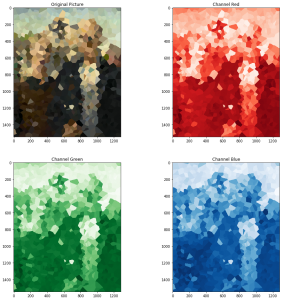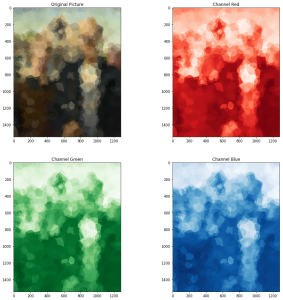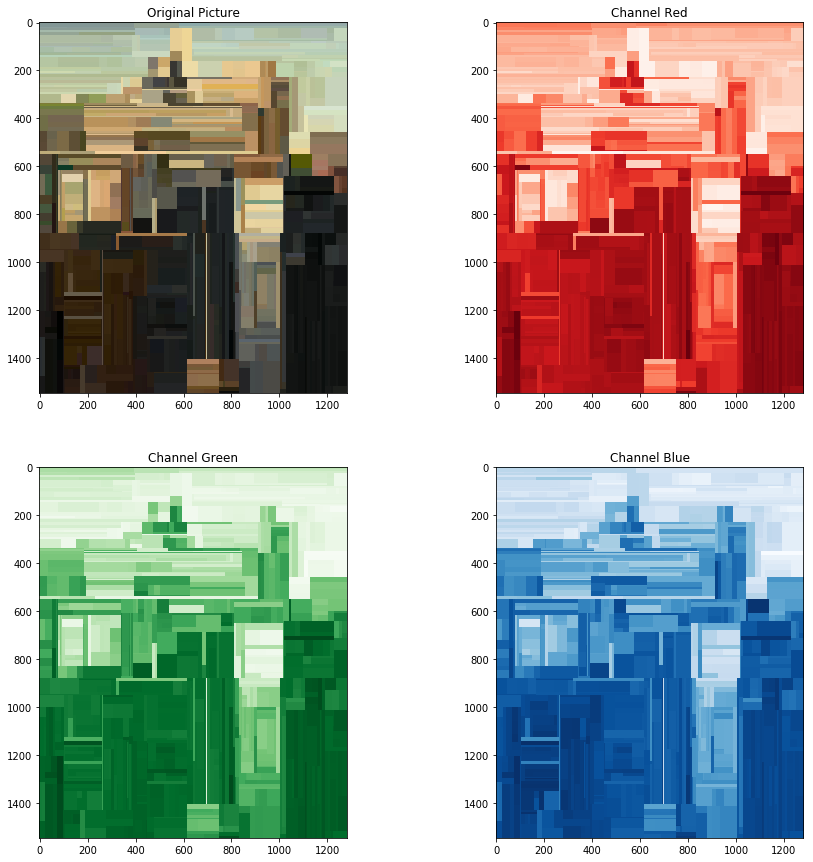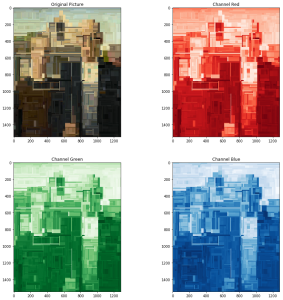After trying regression using linear and SVR models, I wanted to try other two methods offered by scikit-learn based on different principles: K nearest neighbor and decision trees.

# Nearest Neighbors

K-nearest neighbor in this case is straightforward, with K=1 transforms the picture in a mosaic (a Voronoi diagram based on the sampled points):Nearest neighbor model with K=1 and 1000 samples

increasing the value of K, the model will use more points to predict the color of each pixel, doing an average and as a consequence smoothing the zones:Nearest neighbor model with K=3 and 1000 samples

an interesting characteristic of these models is how the points are indexed for faster retrieval during the prediction. When there are two features like in this case, a KD-tree is fine. It generates a tree of zones in the coordinate space where each node is a square (or a cube), to find the closest point in $$log(N)$$ time where $$N$$ is the number of indexed samples. Unlike the R-tree used by spatial databases this index has no overlapping zones and cover the whole space.

When the number of dimensions grow, however, such an index doesn’t work anymore because of the so called curse of dimensionality: the number of ways a point can be close to another grow exponentially increasing the dimension, and it becomes harder for an index to easily exclude a point from the search as there are more and more dimensions it could “use” to be closer. For this scenario, another index technique called ball tree exists and is implemented in scikit-learn; ball tree indexes generate a hierarchy of hyperspheres instead of splitting the space using planes, so can deal with a high number of dimensions. The downside is the increased computational effort during the building of the index.

# Decision trees

Decision trees are one of my favorite models. They are fast and simple, but most importantly the way they make classifications is easy to explain and visualize, to the point the whole model parameters can be printed out.

These are the results of such a model trained on 1000 and 3000 samples from the imageDecision tree trained on 1000 samplesDecision tree trained on 3000 samples

I particularly like the aesthetic of the result using decision trees!